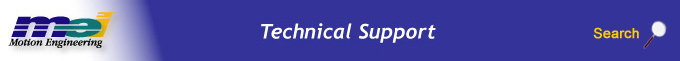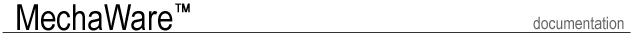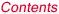.Select a Step ......................................................... Introduction Features User Interface Requirements Types of MechaWare Blocks Block Simulink Library Matlab Utility Software Tools Case Study Tutorial

Control algorithms are specified using a set library of basic building blocks. Once the Filter Object control law is defined, the individual blocks will be converted to the firmware code. The table below shows the list of MEI MechaWare Blocks.

 Actual Position FF 1 PIV 1 Axis Gain 1 Scope 0 BiQuad 1 Integrator 1 SP_BiQuad 2 Clip 1 Matrix Gain Subsystem Command Position 1 Noise 1 Sum 2 Constant OBS Sum 3 Cosine OBS 2 Sum 4 Differentiator 1 Output 1 Sum 5 Error 1 PID 1 TCVelActual Position

An input block, allowing the actual position of any Axis to be used as an input to the control algorithm. The Axis number is specified in the Block Parameters window. Enter ACTUALx for the axis number of the actual position, where x is the axis number. The Block Parameters window is opened by double clicking on the Actual Position Block.Axis

An input block, allowing any Axis to be used as a reference input to the control algorithm. The Axis block is currently only used with the FF block.The Computational Block, implements a second biquad block. Biquad coefficients are specified using a Computation Block Data File. In the simulink environment the biquad block is represented as a second order block only. The firmware does allow for additional cascaded sections to be included in the data file, if desired.

If you are using a ZMP controller, use BiQuad since the ZMP supports double precision math.

If you are using an XMP controller, use SP_BiQuad since the XMP supports single precision math.Clip 1

The clip provides symmetrical limits for a signal. The signal will saturate at a maximum value of (+ Limit) and a minimum value of (– Limit). In addition, an offset can also be added.Command Position 1

An input block, allowing the command position of any Axis to be used as an input to the control algorithm. The Axis number is specified in the Block Parameters window. Enter COMMANDx for the axis number of the command position, where x is the axis number. The Block Parameters window is opened by double clicking on the Actual Position Block.Constant

Constant is a constant number used in an algorithm. A constant would used to feed an input of another block. Double click on the constant block to open the Block Parameters window. Enter a floating point number for the Value in the Block Parameters window.CosineDifferentiator 1

The Differentiator block approximates differentiation using Euler's Backwards Difference Method. Smoothing of the derivative estimate is provided with a first order digital low pass filter with unity gain. The parameter Ksm specifies the filter's breakpoint. Allowable values of Ksm are 0 to 1. Ksm is the discrete pole of the filter.Error 1

An input block, allowing the position error of any Axis can be used as an input to the control algorithm. The Axis number is specified in the Block Parameters window. Enter ERRORx for the axis number of the position error, where x is the axis number. The Block Parameters window is opened by double clicking on the Error Block.FF (Feed Forward) 1

The Feedforward block can be used to add feedforward terms to the control algorithm. There are four feedforward gains:
Kaff: Acceleration Feedforward. Adds a signal proportional to the command acceleration.
Kvff: Velocity Feedforward. Adds a signal proportional to the command velocity.
Kpff: Position feedforward. Adds a signal proportional to the command position.
Kfff: Friction Feedforward. Adds a constant which depends on command direction.Gain 1

Scalar multiplication of input signal.Integrator 1

Integration of input signal using a first order difference equation.Matrix GainNoise 1

The noise block is an input block. The noise block provides access to the XMP/ZMP's internally generated random noise source. The block output is the noise source output multiplied by Kn1. Kn1 and Axis are currently not used.OBS

Mechaware Observer (OBS) block is a general purpose state space implementation of a 2 input, 1 output system of first order difference equations. The order of the specified system can be up to 16. Observer blocks can be incorporated in a variety of applications including, state observation and state feedback, disturbance observation, and system simulation.OBS 2

Mechaware Observer (OBS) block is a general purpose state space implementation of a 2 input, 1 output system of first order difference equations. The order of the specified system can be up to 16. Observer blocks can be incorporated in a variety of applications including, state observation and state feedback, disturbance observation, and system simulation.Output 1

The output block, which allows the filter output to be directed and used by multiple motors in the system.PID 1

This is a standard PID algorithm as used on the XMP. The input (in1) should connect to a position error block or equivalent. The output (Out1) is the torque command ready to connect to a motor output or equivalent. Double click on the PID block to open the Block Parameters window. The coefficients for the PID block are as follows:

 Kp Proportional gain. Kp output = input * Kp Ki Integral gain. Ki output = Σ(input) * Ki, not to exceed IMax Kd Derivative gain. Kd output = (position error (current) - position error (sample - (DRate + 1))) * Kd IMax - Maximum integral output. The integral portion of the PID algorithm will not exceed IMax. Units are in control output counts (+/- 32767) DRate Derivative sub sampling. The derivative will be evaluated every DRate + 1 samples. DRate is not to exceed 7. Keep in mind doubling the derivative evaluation time (DRate = 1 vs. 3, for example) will double the effective derivative gain because of the increase derivative delta time. Ts Sample period of the PID algorithm. This is the cycle time of the PID algorithm in Simulink simulation. This value has no effect on the PID execution on the controller.PIV 1Scope 0

Scope outputs send the value on the scope input to the user buffer. Use the scope to write data every servo sample to memory that is accessible by your program. The scope block will automatically get its own number that corresponds to the user buffer index. For instance, Scope 3 will write its value to User Buffer.If you are using an XMP controller, use SP_BiQuad since the XMP supports single precision math.

If you are using a ZMP controller, use BiQuad since the ZMP supports double precision math.SubsystemSum 2

Sum 2 adds the two inputs to the Sum 2 block and sends them to the output. You can change the gains of the inputs in the Block Parameters window. To open the Block Parameters window, double click on the Sum 2 block.
k1 - the gain for the upper input.
k2 - the gain for the lower input.Sum 3

Sum 3 adds the two inputs to the Sum 3 block and sends them to the output. You can change the gains of the inputs in the Block Parameters window. To open the Block Parameters window, double click on the Sum 3 block.
K1 - the gain for the upper input.
K2 - the gain for the second input.
K3 - the gain for the third input.Sum 4

Sum 4 adds the two inputs to the Sum 4 block and sends them to the output. You can change the gains of the inputs in the Block Parameters window. To open the Block Parameters window, double click on the Sum 4 block.
K1 - the gain for the upper input.
K2 - the gain for the second input.
K3 - the gain for the third input.
K4 - the gain for the fourth input.Sum 5

Sum 5 adds the two inputs to the Sum 5 block and sends them to the output. You can change the gains of the inputs in the Block Parameters window. To open the Block Parameters window, double click on the Sum 5 block.
K1 - the gain for the upper input.
K2 - the gain for the second input.
K3 - the gain for the third input.
K4 - the gain for the fourth input.
K5 - the gain for the fifth input.TcVel

An input block, allowing the Trajectory Calculator's Velocity of any Axis to be used as an input to the control algorithm. The Axis number is specified in the Block Parameters window. Enter AXISx for the axis number of the Trajectory Calculator Velocity, where x is the axis number. The Block Parameters window is opened by double clicking on the TcVel Block.Legal Notice  |  Tech Email  |  Feedback        Copyright © 2001-2009 Motion Engineering